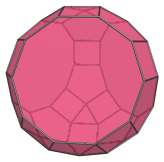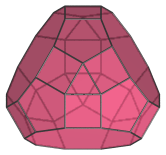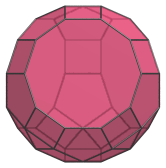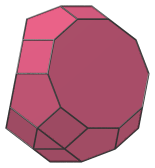# The Tridiminished Rhombicosidodecahedron

The tridiminished rhombicosidodecahedron (J83), also known by its Bowers Acronym tetrid, is the 83rd Johnson solid. It has 45 vertices, 75 edges, and 32 faces (5 triangles, 15 squares, 9 pentagons, 3 decagons). It can be constructed by removing 3 pentagonal cupolae from the rhombicosidodecahedron such that 3 decagonal faces are formed.Due to the non-equivalence of its vertices, the tridiminished rhombicosidodecahedron only has a single axis of symmetry: a 3-fold symmetry around the line passing through the top and bottom triangular faces.

It is a diminishing of the rhombicosidodecahedron in an analogous way to the tridiminished icosahedron (J63) being a diminishing of the icosahedron.

## Projections

In order to be able to identify the tridiminished rhombicosidodecahedron in various projections of 4D objects, it is useful to know how it appears from various viewpoints. The following are some of the viewpoints that are commonly encountered:

Projection DescriptionTop view, showing trigonal symmetry.Front view, perpendicular to top and bottom triangles.Side view, with many coincident edges.

Left long edge is image of a decagon; decagonal face is image of other 2 decagons.

## Coordinates

Cartesian coordinates for the tridiminished rhombicosidodecahedron can be obtained in at least two different ways: by deleting vertices from the rhombicosidodecahedron, or by constructing a series of appropriately-scaled triangles and hexagons on parallel hyperplanes along its 3-fold axis of symmetry.

The following coordinates are obtained the second way, and yield a J83 in a nice orientation, with its axis of symmetry parallel to the Z axis, and having edge length 2:

 (±1, −1/√3, −(4φ+1)/√3) (0, 2/√3, −(4φ+1)/√3) (±φ2, 1/(φ√3), −(2φ+3)/√3) (±φ, (φ+2)/√3, −(2φ+3)/√3) (±1, −φ3/√3, −(2φ+3)/√3) (±1, (2φ+3)/√3, −(2φ+1)/√3) (±(φ+2), −φ/√3, −(2φ+1)/√3) (±φ2, −(φ+3)/√3, −(2φ+1)/√3) (±(2φ+1), −(2φ−1)/√3, −1/√3) (±1, (4φ+1)/√3, −1/√3) (±2φ, −2φ2/√3, −1/√3) (±φ3, −1/√3, (2φ−1)/√3) (±φ, (3φ+2)/√3, (2φ−1)/√3) (±φ2, −(3φ+1)/√3, (2φ−1)/√3) (±1, −(2φ+3)/√3, φ3/√3) (±(φ+2), φ/√3, φ3/√3) (±φ2, (φ+3)/√3, φ3/√3) (±2φ, −2φ/√3, φ3/√3) (0, 4φ/√3, φ3/√3) (±φ2, −1/(φ√3), (2φ+3)/√3) (±φ, −(φ+2)/√3, (2φ+3)/√3) (±1, φ3/√3, (2φ+3)/√3) (±1, 1/√3, (4φ+1)/√3) (0, −2/√3, (4φ+1)/√3)

where φ=(1+√5)/2 is the Golden Ratio.

## Occurrences

The tridiminished rhombicosidodecahedron appears as cells in the following CRF polychora, generalizations of the Johnson solids to 4D:

Last updated 25 Feb 2019.